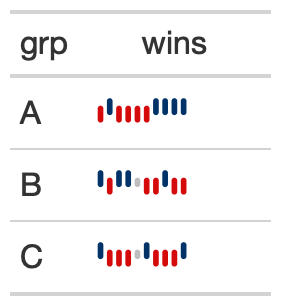The gt_plt_winloss function takes an existing gt_tbl object and adds squares of a specific color and vertical position based on wins/losses. It is a wrapper around gt::text_transform(). The column chosen must be a list-column as seen in the example code. The column should also only contain values of 0 (loss), 0.5 (tie), and 1 (win).

## Usage

gt_plt_winloss(
gt_object,
column,
max_wins = 17,
palette = c("#013369", "#D50A0A", "gray"),
type = "pill",
width = max_wins/0.83
)

## Arguments

gt_object

An existing gt table object of class gt_tbl

column

The column wherein the winloss plot should replace existing data. Note that the data must be represented as a list of numeric values ahead of time.

max_wins

An integer indicating the max possible wins, this will be used to add padding if the total wins/losses observed is less than the max. This is useful for mid-season reporting. Defaults to a red, blue, grey palette.

palette

A character vector of length 3, specifying the colors for win, loss, tie in that exact order.

type

A character string representing the type of plot, either a 'pill' or 'square'

width

A numeric indicating the width of the plot in mm, this can help with larger datasets where data points are overlapping.

## Value

An object of class gt_tbl.

## Examples

#' library(gt)

set.seed(37)
data_in <- dplyr::tibble(
grp = rep(c("A", "B", "C"), each = 10),
wins = sample(c(0,1,.5), size = 30, prob = c(0.45, 0.45, 0.1), replace = TRUE)
) %>%
dplyr::group_by(grp) %>%
dplyr::summarize(wins=list(wins), .groups = "drop")

data_in

win_table <- data_in %>%
gt() %>%
gt_plt_winloss(wins)## Function ID

3-1

Other Plotting: gt_plt_bar_pct(), gt_plt_bar_stack(), gt_plt_bar(), gt_plt_dist(), gt_plt_percentile(), gt_plt_point(), gt_plt_sparkline()